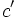# Black-box group algorithm for fixed-class nilpotency testing given a generating set

## Summary

Item Value
Problem being solved fixed-class nilpotency testing problem: determine whether a group is nilpotent of a given nilpotency class, i.e., the nilpotency class is at most equal to a specified value.
Input format a finite group$G$ of order$N$ given via an encoding of a group. A positive integer$c$. A generating set$S$ of size$s$ for$G$.
Output format Yes/No depending on whether$G$ has nilpotency class at most$c$.
In the case of a No answer, can also output a tuple of length$c + 1$ with letters from$S$ whose iterated left-normed commutator is not the identity element.
Running time$\! O(s^{c' + 1}c')$ times the time for group operations. Here$c' = \min \{ c, \log_2N\}$ if we know$N$, otherwise simply set$c' = c$. For small values of$c$,$c' = c$.
Black-box group operations used group multiplication, inverse map, and identity element. This algorithm does not use the membership test.
for a multi-encoding, also needs equality testing.
Parallelizable version The computations of iterated commutators can be massively parallelized, if all the parallel processes can simultaneously access the algorithms for the group operations.

## Idea and outline

First, note that if a group of order$N$ is nilpotent, its nilpotency class is at most$\log_2N$ (in fact, it is at most$\log_2N - 1$). Thus, checking if the group has nilpotency class at most$c$ is equivalent to checking if the group has nilpotency class at most$c'$ where$c' = \min \{ c, \log_2N \}$. This is useful if we know$\log_2N$. If we do not, we simply use$c' = c$.

To check this, it suffices to verify that all iterated left-normed commutators of length$c + 1$ from elements of$S$ give the identity element.

In practice, we could tweak the algorithm a little bit: run it on every smaller value than$c'$ (i.e., check all commutators of length 2, then all commutators of length 3, ...). Since we're using iterated left-normed commutators, the commutators computed in any one iteration can be used to save time for the next iteration. An advantage of this is that if the group has smaller nilpotency class, the algorithm terminates faster.

## Analysis of running time

For checking each tuple: Each of these commutator computations requires$c' + 1$ commutator computations, and each commutator computation requires three multiplications and one inversion, so we have a total of$O(c)$ group operations. Thus, the time taken to check a tuple is$O(c')$ times the time taken for group operations.

Total time: The total time is$\! s^{c'+1}$ times the time taken for each tuple.